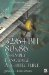General Integer MathWe learned negation with the NEG instruction in Chapter 4, "Bit Mangling," and binary multiplication (2 N ) and division using bit shifting in Chapter 5, "Bit Wrangling."

We can add 8-, 16-, 32-, and 64-bit numbers , and 4-bit if you include BCD (binary-coded decimal) discussed later in Chapter 15.

 No 64-bit The general-purpose BCD instructions are not available in 64-bit mode!

The EFLAGS Overflow, Sign, Zero, Auxiliary Carry, Parity, and Carry are all altered when using the general-purpose instructions.

 Overflow Whether the numbers overflow the binary limit of the destination operand Sign Set to the resulting value of the MSB (most significant bit) Zero Set if the result is a value of zero Aux. Carry Set as a result of a carry of the low-order nibble Parity Set to 1 if the bits add up to an even number; 0 if not Carry Any mathematical carry results of the mathematical operation

ADD destination , source

Mnemonic

P

PII

K6

3D!

3Mx+

SSE

SSE2

A64

SSE3

E64T32/64-Bit 80x86 Assembly Language Architecture
ISBN: 1598220020
EAN: 2147483647
Year: 2003
Pages: 191

Similar book on Amazon